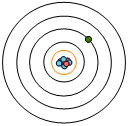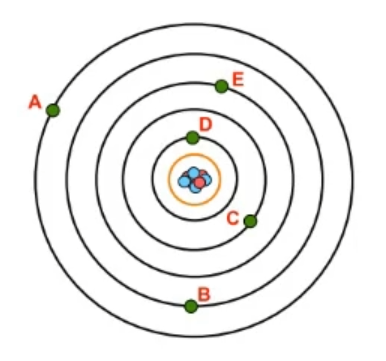Clutch Prep is now a part of Pearson
Ch.7 - Quantum MechanicsWorksheetSee all chapters

# Quantum Numbers: Principal Quantum Number

See all sections
Sections
Wavelength and Frequency
Speed of Light
The Energy of Light
Electromagnetic Spectrum
Photoelectric Effect
De Broglie Wavelength
Heisenberg Uncertainty Principle
Bohr Model
Emission Spectrum
Bohr Equation
Introduction to Quantum Mechanics
Quantum Numbers: Principal Quantum Number
Quantum Numbers: Angular Momentum Quantum Number
Quantum Numbers: Magnetic Quantum Number
Quantum Numbers: Spin Quantum Number
Quantum Numbers: Number of Electrons
Quantum Numbers: Nodes

The Principal Quantum Number gives both the size and energy of an electron shell.

###### Principal Quantum Number

Concept #1: Principal Quantum Number

Example #1: Which of the following is a possible value for a principal quantum number shell?

Practice: What is the value of n for the electron based on the image of the atom provided?Practice: Which electron possesses the lowest possible energy from the image provided?